# Horizontal and Vertical Lines

Horizontal line is a straight line that is mapped from left to right, whereas the vertical is a straight line that is mapped from top to bottom.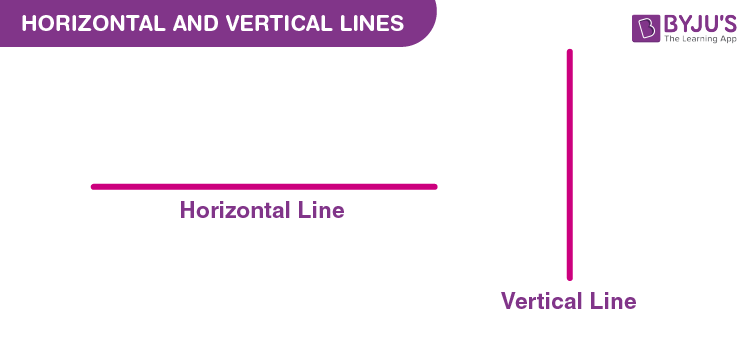In coordinate geometry, the line parallel to the x-axis is called horizontal line and the line which is parallel to the y-axis is called vertical line. Horizontal lines will be perpendicular to y-axis and vertical lines will be perpendicular to x-axis. Thus, horizontal and vertical lines are perpendicular to each other when drawn with at least one common point. This can be shown as: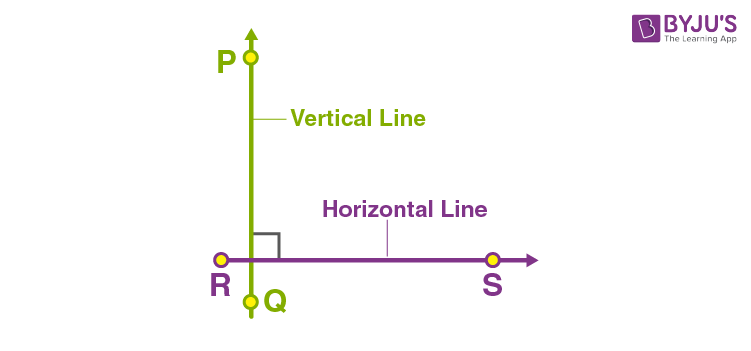## Equations for Horizontal and Vertical Lines

We can derive the equations of vertical and horizontal lines in coordinate geometry. The equation of horizontal lines can be derived with respect to the y-coordinate and the equation of vertical lines can be derived from the x-coordinate of points. These are explained below with suitable examples.

### Equation of Horizontal Line

Suppose a horizontal line L is at a distance “a” from the x-axis. Here, the ordinate of every point lying on the line is either a or – a. Therefore, the equation of the line L is either y = a or y = – a. Choice of the sign will depend upon the position of the line according to whether the line is above or below the x-axis. The below figure shows the horizontal lines y = a and y = -a.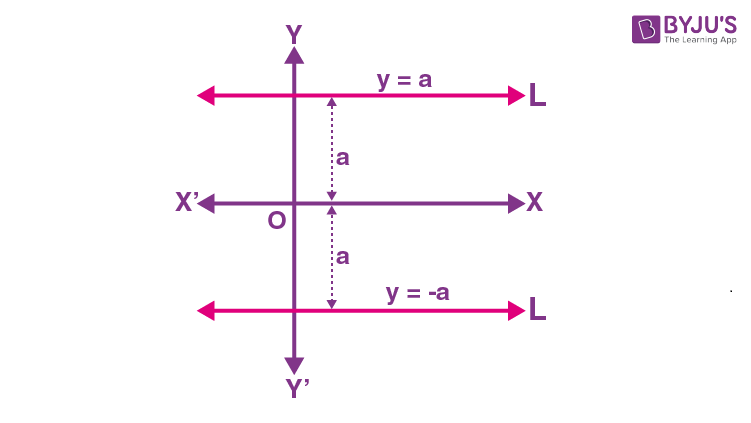### Equation of Vertical Line

Consider a vertical line L is at a distance “b” from the y-axis. Here, the ordinate of every point lying on the line is either b or – b. Therefore, the equation of the line L is either x = b or x = – b. However, the choice of the sign will depend upon the position of the line according to whether the line is on the left or right side of the y-axis. For example, the below figure shows the vertical lines x = b and x = -b.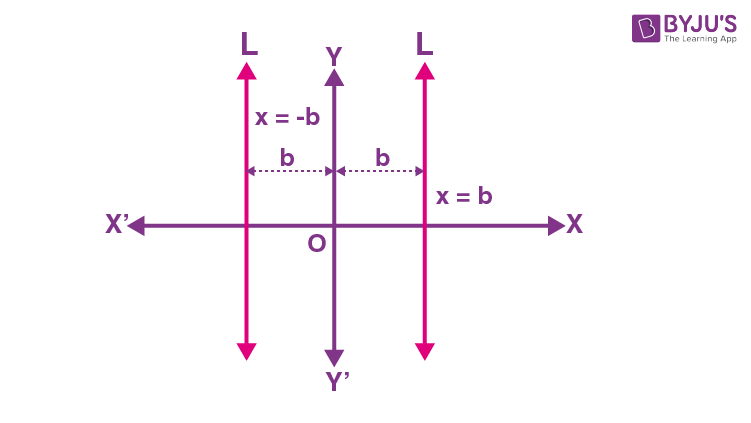### Horizontal and Vertical Lines Images

Below figure shows vertical and horizontal lines separately. These are the basic representations of vertical and horizontal lines. However, we can draw vertical lines and horizontal lines as per the instructions provided and as per the given information in many situations.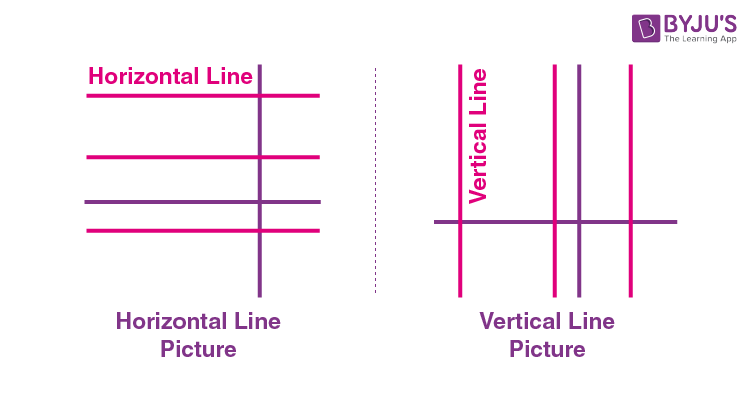### Horizontal and Vertical Lines of Symmetry

We know that we can draw either horizontal, vertical, or diagonal lines of symmetry. If the line of symmetry is parallel to the horizontal plane, then it is known as the horizontal line of symmetry. If the line of symmetry is parallel to the vertical plane, then it is known as the vertical line of symmetry. We can show both types of symmetry in different shapes. These lines divide the shapes into two parts such that they are mirror images to one another. Below are examples of horizontal lines of symmetry.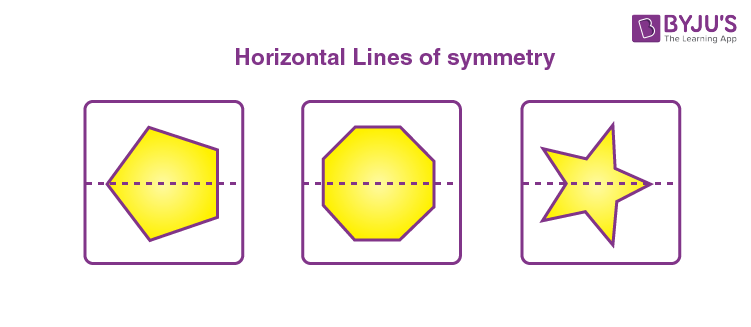Now, have a look at the examples of vertical lines of symmetry.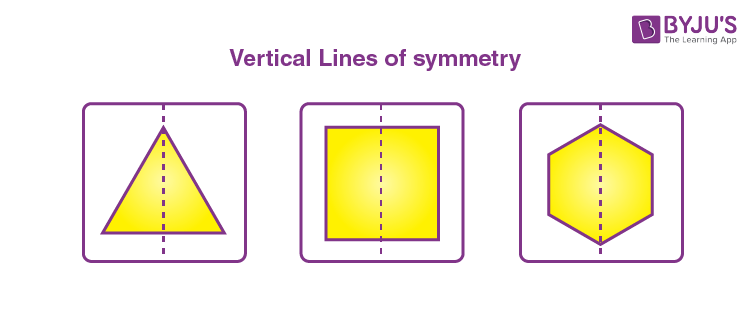For some shapes we can show both horizontal and vertical lines of symmetry such as:### Graphing Horizontal and Vertical Lines

For a point in coordinate space, we can show the horizontal and vertical lines as given below: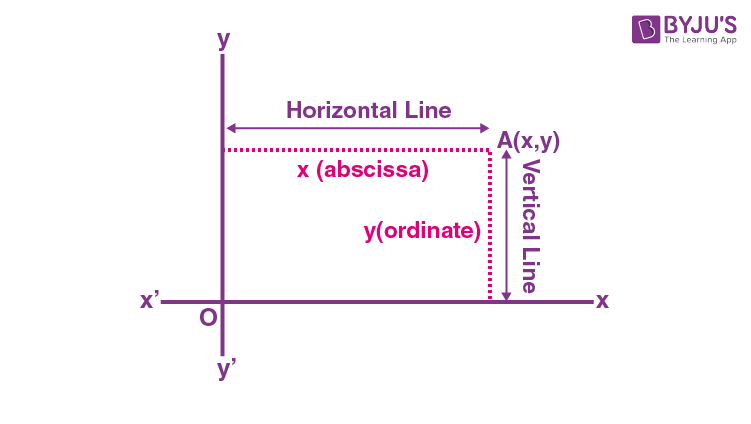Based on this we can draw the lines which may be horizontal or vertical. Go through the example given below to understand how to graph the given point and derive the equations for lines.

Example:

Find the equations of the lines parallel to axes and passing through (4, 2).

Solution:

Given point: (4, 2)

Here,

x-coordinate = 4

y-coordinate = 2

Let us plot this point on the coordinate plane as given below: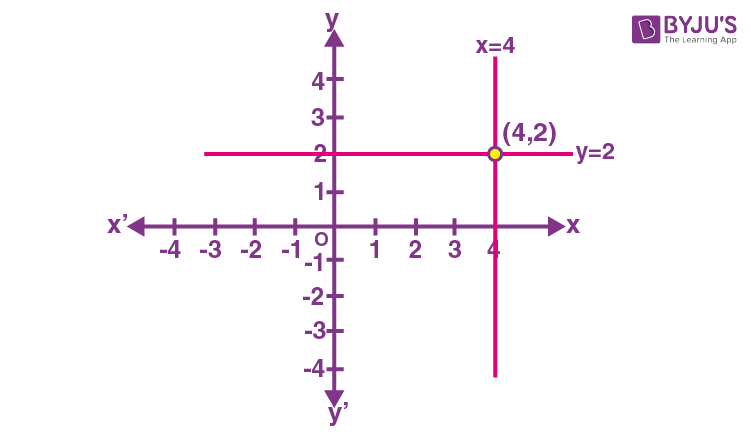The y-coordinate of every point on the line parallel to the x-axis is 2.

Therefore, the equation of the line parallel to x-axis and passing through (4, 2), i.e. horizontal line equation is y = 2.

Similarly, the equation of the line parallel to the y-axis and passing through (4, 2), i.e. vertical line is equation x = 4.

For more such interesting concepts, visit www.byjus.com and download BYJU’S – The Learning App to get visualised explanations for maths concepts.